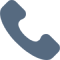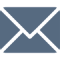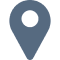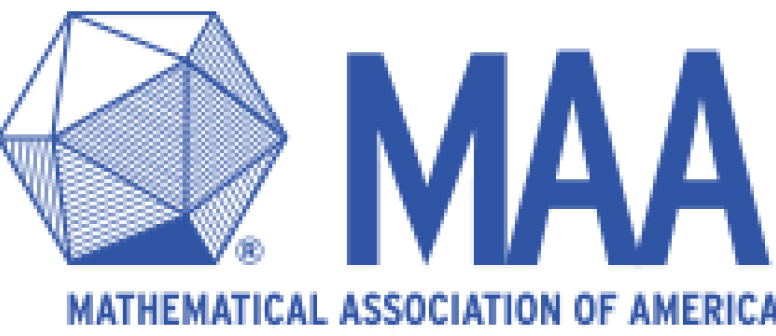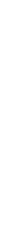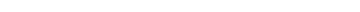# What is the USA(J)MO?

• The USA(J)MO is the national mathematics olympiad, from which top scorers are invited to the Math Olympiad Program (MOP) where the USA IMO team is selected.
• The top roughly 200 participants from AMC 12 and AIME qualify for the USA Mathematics Olympiad (USAMO), while the top roughly 200 participants from the AMC 10 and AIME qualify for the USA Junior Mathematics Olympiad (USAJMO).
• The USA(J)MO is a strenuous 2-day, 9-hour, and 6-problem test of challenging and intensive proof-based problems, which is the same format of testing as the IMO. The USAMO, in particular, rivals and sometimes exceeds the difficulty of the International Mathematical Olympiad.# Syllabus & Schedule

syllabus
• Algebra, Geometry, Number Theory, and Combinatorics (knowledge of calculus in not required but can be helpful at times)
• One of the hardest math contests in the world, extremely strenuous and challenging even for the top minds of the country. Solving even one question is considered to be an accomplishment.
Schedule
• Sometime in mid-late April, a little over a month after the AIME.

• The 2020 USA(J)MO was administered on June 19 and June 20, 2020.# Criteria & Format

The USA(J)MO exam is a 6 problem exam. There are 540 minutes given in the exam. Problems 1/4 are easier than 2/5, which are easier than 3/6.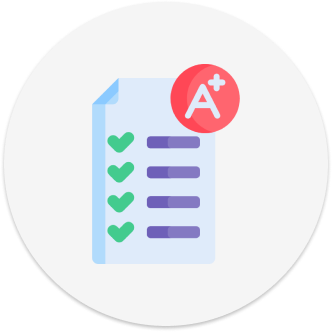Criteria to Qualify
Top scorers in the AMC and AIME are invited to take this exam. Only around 400 contests are invited in total.6 Questions
The USA(J)MO exam is a 6 problem exam.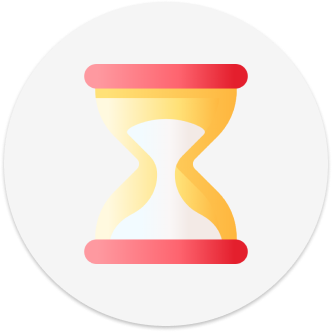540 Minutes
There are 540 minutes given in the exam.

Due to time constraints, and for contestants to perform optimally, the test is split into two days of 270 minutes each.Difficulty
Problems 1/4 are easier than 2/5, which are easier than 3/6.

The USA(J)MO is considered to be one of the hardest math contests to qualify or participate in.

# Results for USA(J)MO

The USA(J)MO  is the second exam in the series of exams used to challenge bright students on the path toward choosing the team that represents the United States at the International Mathematics Olympiad (IMO).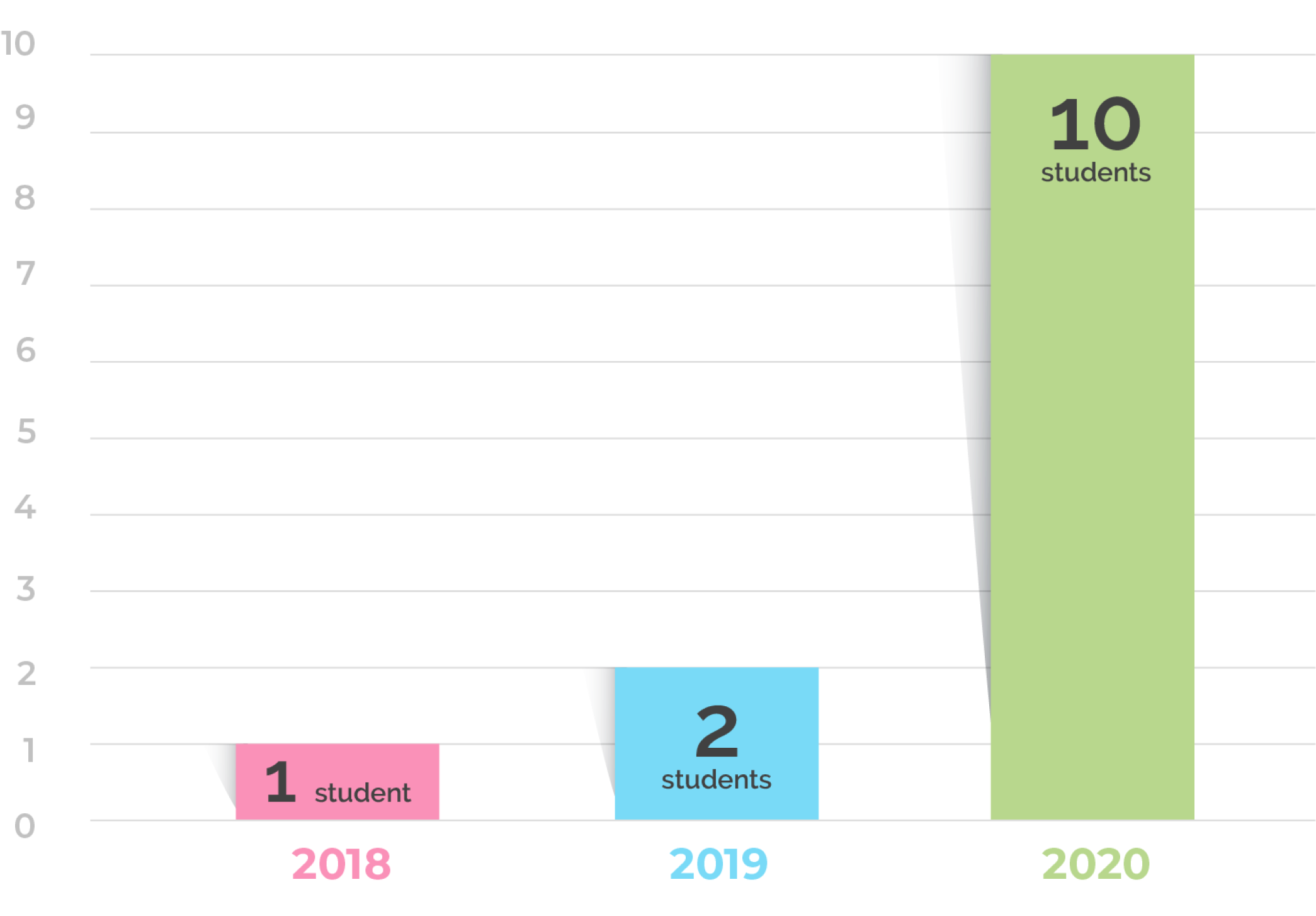10 Random Math USA(J)MO Qualifiers
• This year, 10 students have qualified for USA (Junior) Math Olympiad.
• The USA(J)MO is an intensive test lasting 9 hours in total, split between 2 days. There is a total of 6 proof-based questions to be answered in that time.